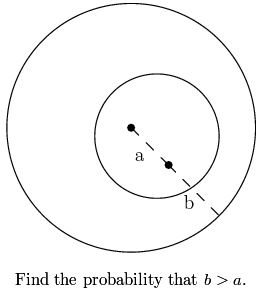Point in a circleA circle of radius 1 is randomly chosen strictly inside a circle of radius 2. A point is chosen, again at random, inside the circle with radius 1. What is the probability this point is closer to the center of the circle with radius 2 than the circumference of the circle with radius 2?Note by Daniel Liu
6 years ago

This discussion board is a place to discuss our Daily Challenges and the math and science related to those challenges. Explanations are more than just a solution — they should explain the steps and thinking strategies that you used to obtain the solution. Comments should further the discussion of math and science.

When posting on Brilliant:

• Use the emojis to react to an explanation, whether you're congratulating a job well done , or just really confused .
• Ask specific questions about the challenge or the steps in somebody's explanation. Well-posed questions can add a lot to the discussion, but posting "I don't understand!" doesn't help anyone.
• Try to contribute something new to the discussion, whether it is an extension, generalization or other idea related to the challenge.
• Stay on topic — we're all here to learn more about math and science, not to hear about your favorite get-rich-quick scheme or current world events.

MarkdownAppears as
*italics* or _italics_ italics
**bold** or __bold__ bold

- bulleted
- list

• bulleted
• list

1. numbered
2. list

1. numbered
2. list
Note: you must add a full line of space before and after lists for them to show up correctly
paragraph 1

paragraph 2

paragraph 1

paragraph 2

> This is a quote
This is a quote
# I indented these lines
# 4 spaces, and now they show
# up as a code block.

print "hello world"
# I indented these lines
# 4 spaces, and now they show
# up as a code block.

print "hello world"
MathAppears as
Remember to wrap math in $$...$$ or $...$ to ensure proper formatting.
2 \times 3 $2 \times 3$
2^{34} $2^{34}$
a_{i-1} $a_{i-1}$
\frac{2}{3} $\frac{2}{3}$
\sqrt{2} $\sqrt{2}$
\sum_{i=1}^3 $\sum_{i=1}^3$
\sin \theta $\sin \theta$
\boxed{123} $\boxed{123}$

Sort by:

Edited to match the corrected question...

Let $R$ be the distance of the centre $O_2$ of the circle $C$ of radius $1$ from the centre $O_1$ of the circle of radius $2$. Since $O_2$ can be anywhere inside the circle of radius $1$ with centre $O_1$ (shown dotted in the diagram) we have $\mathbb{P}[R \le r] \,=\, \tfrac{\pi r^2}{\pi} \,=\, r^2$ for any $0 \le r \le 1$ and hence $R$ has the probability distribution function $f(r) \; = \; \left\{ \begin{array}{ll} 2r & 0 \le r \le 1 \\ 0 & \mbox{o.w.} \end{array} \right.$alternatetext

Given that $R = r$, the probability that the randomly chosen point in the circle $C$ is closer to the centre of the original circle than its circumference is the probability that the randomly chosen point lies inside the region common to $C$ and the (dotted) circle of centre $O_1$ and radius $1$, which is $\begin{array}{rcl} \mathbb{P}[b > a | R = r] & = & \tfrac{2}{\pi}(\theta - \sin\theta\cos\theta) \\ & = & \tfrac{1}{\pi}\left[2\cos^{-1}(\tfrac{r}{2}) - r\sqrt{1 - \tfrac14r^2}\right] \end{array}$ and hence $\begin{array}{rcl} \mathbb{P}[b > a] & = & \displaystyle\int_0^1 \mathbb{P}[b > a|R=r]\,2r\,dr \; = \; \frac{2}{\pi}\int_0^1\left(2r\cos^{-1}(\tfrac{r}{2}) - r^2\sqrt{1 - \tfrac14r^2}\right)\,dr \\ & = & \displaystyle\frac{2}{\pi}\int_{\frac12\pi}^{\frac13\pi}\left(4u\cos u - 4\cos^2u\sin u\right)(-2\sin u)\,du \\ & = & \displaystyle\frac{2}{\pi}\int_{\frac13\pi}^{\frac12\pi}\left(4u\sin2u - 2\sin^22u\right)\,du \\ & = & \displaystyle\frac{2}{\pi}\int_{\frac23\pi}^\pi \left(v\sin v - \sin^2v\right)\,dv \\ & = & \displaystyle\frac{2}{\pi}\left[ -v\cos v + \sin v - \tfrac12v + \tfrac14\sin2v\right]_{\frac23\pi}^\pi \\ & = & \frac{2}{\pi} \times \frac{1}{8}(4\pi - 3\sqrt{3}) \; = \; 1 - \frac{3\sqrt{3}}{4\pi} \end{array}$

- 6 years ago

Hi,

I tried out a simulation, choosing the coordinates $(r_1\, \cos \theta+r_2\, \cos \alpha ,r_1\, \sin \theta+r_2\, \sin \alpha )$ at random, where $0\le r_1, r_2 \le 1$ and $0\le \theta, \alpha \le 2\,\pi$.

The distance from the center of the bigger circle, $a=\sqrt{r_1^2+r_2^2+2\, r_1\, r_2\, \cos {(\theta-\alpha)}}$ and $b = 2 -a$, simulating for 1000000 trials, I got the average $b > a$ about 0.777, which is more than 0.5865.

Can't see any mistake, though.

- 6 years ago

Did you assume that $r_1$ and $r_2$ were uniform on $[0,1]$?

- 6 years ago

Yes, I did..

- 6 years ago

The pdf of $r_1$ and $r_2$ is $2x$ for $0\le x \le 1$. Look at the beginning of my solution.

- 6 years ago

I saw, but I thought it was required only for deriving the answer analytically.

$r_1$ is the distance from the center of the circle with radius 2 centered at $(0,0)$, and get the coordinates $(r_1\cos \theta, r_1 \sin \theta )$, which is the center of the smaller circle. Then select $(r_2\cos \alpha, r_2 \sin \alpha )$, which is the point within the smaller circle. Then I did my simulation as in the earlier post.

Is there something wrong with this thinking? Aren't the variables independent?

- 6 years ago

If you are going to simulate this, you must adjust your simulation so that the chances of choosing $r_1$ and $r_2$ correct. You can ignore $\theta$. Provided that you choose $r_1$ and $r_2$ independently from the correct pdf, and choose $\alpha$ randomly and uniformly in $[0,2\pi)$, all should be well. It will not work if you choose $r_1$ and $r_2$ randomly from a uniform distribution.

To model $r_1$ and $r_2$ correctly, choose $r_1^2$ and $r_2^2$ from a uniform distribution on $[0,1]$. If you do that, your simulation should agree with my theoretical result (my one did).

- 6 years ago

Oh, I see! It's agreeing now.

Thanks for your help, much appreciated.

- 6 years ago

Sorry, little typo there. Fixed. That would mean the probability is $\dfrac{4\pi-3\sqrt{3}}{4\pi}$, correct? I never thought this problem would actually be that hard.

- 6 years ago